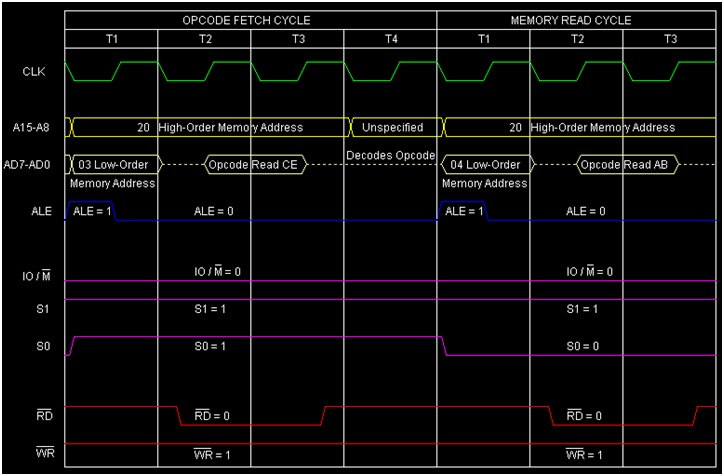# Instruction type ACI d8 in 8085 Microprocessor

In 8085 Instruction set, ACI is a mnemonic which stands for 'Add with Carry Immediate to Accumulator' and here “d8” stands for any 8-bit or 1-Bytedata. This instruction is actually meant for adding one 8-bit immediate data or operand to the Accumulator along with the carry value. The result of the addition will be stored in the Accumulator itself and replacing initial value of the Accumulator. As it is an arithmetic instruction, so the flags are affected based on the result. It holds 2-Bytes in the memory.

Mnemonics, Operand Opcode(in HEX) Bytes
ACI Data CE 2

Let us consider one sample instruction ACI ABH falling in this category. It is a 2-Byte instruction, so it will occupy two consecutive memory locations to hold the instruction.Let us consider the initial content of the Accumulator is 10H. So operand ABH with carry will be added with the Accumulator. Let the initial carry is 1. So the final content of the Accumulator will be 10H + ABH + 1 = BCH. The result of execution of this instruction is depicted with the following tracing table.

Before After

(A)

10H BCH

(F)

Cy=1 Other flag bits=any values Cy=0,AC=0,S=1,P=0,Z=0